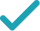Type a math problemSolve for xSolution Steps
Multiply both sides of the equation by , the least common multiple of . Since is , the inequality direction remains the same.
Use the distributive property to multiply by .
Use the distributive property to multiply by .
Subtract from both sides.
Combine and to get .
GraphGraph Inequality
Graph Both Sides in 2DGiving is as easy as 1, 2, 3
Get 1,000 points to donate to a school of your choice when you join Give With Bing
3\left(x-2\right)\geq 2\left(x-1\right)+7
Multiply both sides of the equation by 18, the least common multiple of 6,9,18. Since 18 is >0, the inequality direction remains the same.
3x-6\geq 2\left(x-1\right)+7
Use the distributive property to multiply 3 by x-2.
3x-6\geq 2x-2+7
Use the distributive property to multiply 2 by x-1.
3x-6\geq 2x+5
Add -2 and 7 to get 5.
3x-6-2x\geq 5
Subtract 2x from both sides.
x-6\geq 5
Combine 3x and -2x to get x.
x\geq 5+6